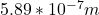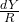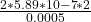## In a double slit interference experiment, the distance between the slits is 0.0005m and the screen is 2 meters from the slits. Yellow light

Question

In a double slit interference experiment, the distance between the slits is 0.0005m and the screen is 2 meters from the slits. Yellow light from a sodium lamp is used and it has a wavelength of 5.89 x 10-7 m. What is the distance between the first and second bright fringes on the screen?

in progress 0
6 months 2021-07-20T03:06:00+00:00 1 Answers 13 views 0

## Answers ( )

4.712 mm or 0.004712 m

Explanation:

Parameters given:

Distance between the slits, d = 0.0005 m

Distance between the screen and the slits, R = 2 m

Wavelength of the light, λ =The condition for the maxima in a double slit experiment is given as:

mλ =where Y = distance between the first order maxima and the mth order maxima

In this case, m = 2.

Therefore:

Y = mλR/d

Y =Y = 0.004712 m = 4.712 mm

The distance between the first and second bright fringes is 4.712 mm.